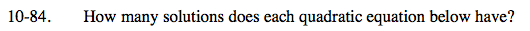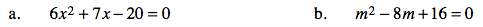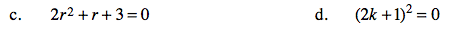### Home > AC > Chapter 10 > Lesson 10.2.4 > Problem10-84A

10-84A.Factor the equation.

Since the two factors are not equal, there are two solutions.

Factor the equation.

Since the two factors are equal, there is one solution.This quadratic cannot be factored. Use the Quadratic Formula to deterine how many solutions this equation has.

If he number under the square root is greater than 0, then there are two solutions. If the number under the square root is equal to 0, then there is only one solution.

Refer to parts (a) and (b).